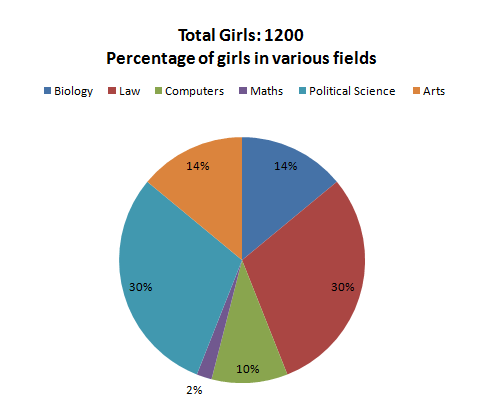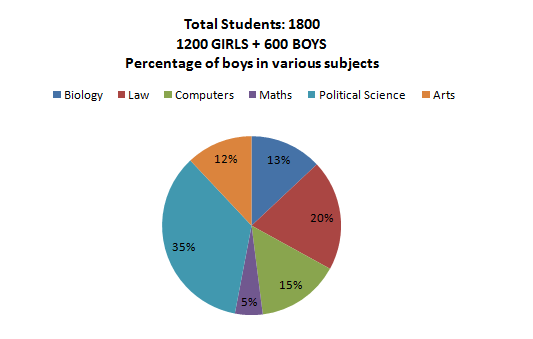Directions: Study the following pie-charts carefully to answer these questions.

The graphs depict the following:

• Percentage of students in a college studying various subjects
• Percentage of girls out for various subjects.Question 1: The number of girls studying Arts in college is

(a) 242
(b) 168
(c) 120
(d) 276

Question 2: For which subject is the number of boys the minimum?
(a) Law
(b) Biology
(c) Arts
(d) Maths

Question 3: For Political Science, what is the ratio of boys and girls?
(a) 4 : 3
(b) 3 : 4
(c) 2 : 3
(d) 4 : 5

Question 4: The number of girls studying Arts is what percent more than the number of boys studying Arts?
(a) 170%
(b) 150%
(c) 80%
(d) 250%

The required number = 14% of 1200 = 168.

The correct option is (b)

In Law, the number of boys = 20% of 1800 – 30% of 1200 = 360 –360 = 0.

Thus, the number of boys in law is least.

The correct option is (a)

The required ratio is

= {(35% of 1800 – 30% of 1200)/(30% of the 1200)}

= ((630 -360)/360}

=27/36 = 3:4

The correct option is (b)

Number of girls studying arts = 14% of 1200 = 168

Number of boys studying arts = 12% of 1800 -168 = 216-168 = 48

The required percentage = {(168 – 48)/48} x 100 = 120/48 x 100 = 250%

### Want to explore more Data Interpretation Sets?

Get Posts Like This Sent to your Email
Updates for Free Live sessions and offers are sent on mail. Don't worry: we do not send too many emails..:)
Get Posts Like This Sent to your Email
Updates for Free Live sessions and offers are sent on mail. Don't worry: we do not send too many emails..:)